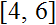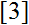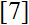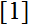# Volume 12, 2006, Number 4

Volume 12Number 1Number 2Number 3 ▷ Number 4

Generalized Fibonacci and Lucas sequences with Pascal-type arrays
Original research paper. Pages 1—9
Charles K. Cook and A. G. Shannon
Full paper (53 Kb) | Abstract

We re-label the Fibonacci and Lucas sequences respectively by
{F0,n} ≡ {Fn} and {F1,n} ≡ {Ln}
and consider
Fm,n = Fm−1, n−1 + Fm−1, n+1, m, n ≥ 1,
as a generalization of the well-known identity
Ln = Fn−1 + Fn+1,
where
Fm,n = Fm,n−1 + Fm, n−2, m ≥ 1, n > 2.

On Steiner Loops of cardinality 20
Original research paper. Pages 10—22
M. H. Armanious
Full paper (184 Kb) | Abstract

It is well known that there are five classes of sloops of cardinality 16 ” SL(16)s” according to the number of sub-SL(8)s. In this article, we will show that there are exactly 8 classes of nonsimple sloops and 6 classes of simple sloops of cardinality 20 “SL(20)s”. Based on the cardinality and the number of (normal) subsloops of SL(20), we will construct in section 3 all possible classes of nonsimple SL(20)s and in section 4 all possible classes of simple SL(20)s. We exhibit the algebraic and combinatoric properties of SL(20)s to distinguish each class.

So we may say that there are six classes of SL(20)s having one sub-SL(10) and n sub-SL(8)s for n = 0, 1, 2, 3, 4 or 6. All these sloops are subdirectly irreducible having exactly one proper homomorphic image isomorphic to SL(2). For n = 0, the associated SL(20) is a nonsimple subdirectly irreducible having one sub-SL(10) and no sub-SL(8)s. Indeed, the associated Steiner quasigroup SQ(19) of this case supplies us with a new example for a semi-planar SQ(19), where the smallest well-known example of semi-planar squags is of cardinality 21 ” cf.“.

It is well known that there is a class of planar Steiner triple systems (STS(19)s) due to Doyen, where the associated planar SL(20) has no sub-SL(10) and no sub-SL(8). In section 4 we will show that there are other 6 classes of simple SL(20)s having n sub-SL(8)s for n = 0, 1, 2, 3, 4, 6, but no sub-SL(10)s. It is well-known that a sub-SL(m) of an SL(2m) is normal. In the last theorem of this section, we give a necessary and sufficient condition for a sub-SL(2) to be normal of an SL(2m). Accordingly, we have shown that if a sloop SL(20) has a sub-SL(10) and 12 sub-SL(8), then this sloop is isomorphic to the direct product SL(10) × SL(2) and if a sloop SL(20) has 12 sub-SL(8)s and no sub-SL(10), then this sloop is a subdirectly irreducible having exactly one proper homomorphic image isomorphic to SL(10). In section 5, we describe how can one construct an example for each class of smiple and of nonsimple SL(20)s.

Note on φ, ψ and σ functions
Original research paper. Pages 23—24
Krassimir T. Atanassov
Full paper (95 Kb) | Abstract

In the present remark we shall formulate and discuss some extremal problems, related to arithmetic functions φ, ψ and σ (see, e.g.,).

Volume 12Number 1Number 2Number 3 ▷ Number 4